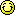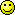### Welcome to Chow Ka Fat's Forum

You are welcome to post messages in this forum. But please observe the rules of this forum. Any messages violating the rules will be deleted and the person posting such messages may be forbidden to post messages.

Welcome to Chow Ka Fat's Forum
Author
Comment
Maths question

I have a Maths question to ask you
1＊２＊３＊４＊５．．．．．＊１００

Re: Maths question

Re: Maths question

100!=4
1*2*3*4*5*6*7*8*9*10
11*12*13*14*15*16*17*18*19*20
...
91*92*93*94*95*96*97*98*99*100

thus
by ignoring zero, considering the last digit only:(1*2*3*4*5*6*7*8*9) repeat 10 times
1*2*3*4*5*6*7*8*9=8=2^3
repeat 10 times
2^30

(since
2*2=4
2*4=8
8*2=16
16*2=32=2^5

thus)
2^30=((2^4)^7)*2^2=4

Re: Maths question

thank youRe: Maths question

I find a 公式(only for 100 倍數)
24x(n/100)+( (n/100)-1)Re: Maths question

Re: Maths question

Dear friends,

My (F.3 promote to F.4) son's has a few questions of his summer homework. Because he fears Maths so much
and seldom pass. He lacks confidence now.

Pls would you tell me the solution to these
questions, so that I might help him. Thank's
very much for any advice you could give :

1. simplify the following expressions.
___________________
a. / 0.00025 x 49000
___
b. 9 +/ 50
___ ____
__
/8 5

__ __
2.If/2 = m and /3 = n, express the following
in terms of m and n.

___
a./18

______
b./0.03

____
c./0.5

____
d./1200

3. Simplify 1/x - 1/x-1

answer a. 1/x(x-1), b. 1/x(1-x), c. 2x-1/ x(x-1),
d. 2x-1 / x(1-x)

5. If x+y /x-y = y, then x =

a. y(y+1) / y-1, b. y(y-1)/y+1, c. y+1/y(y-1),
d. y-1/ y(y+1)

Re: Maths question

Dear Mrs. Chan,

Here I provide some hints which may be helpful to you.

For 1a, you can first rewrite 0.00025 as 25 x 10-4 and the same for 49000. Then you should be able to simplify the expression. Remember that the square root of 10-4 is 10-2.

For 1b, I don't quite understand the expression because there are some lines. Does it represent a fraction?

For 2, I would show you the solution to part (a) as an example. Since 18 = 2x9, /18 = 3x /2 = mn2. For the other parts, you just use the same principle. Please note that 2 = m2 and 3 = n2. For part (c), please remember 0.5 = 1/2.

For 3, you should use the method of "通分母" to simplify the expression.

For 4, you first multiply both sides by x-y to get x+y = y(x-y). Then try to put the terms with x on one side and then factorize. Then you should be able to find x in terms of y.